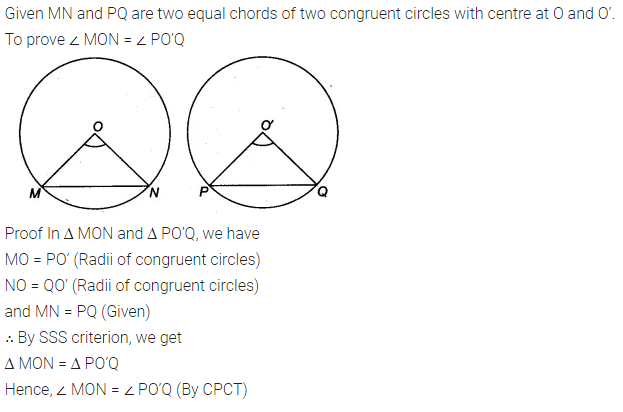# Prove that equal chords of congruent circles subtend equal angles at their centres

Recall that two circles are congruent, if they have the same radii. Prove that equal chords of congruent circles subtend equal angles at their centres